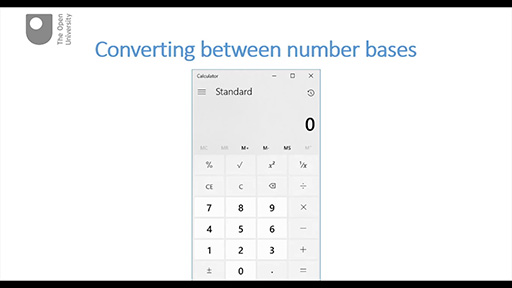Digital & Computing

### Become an OU studentDiscovering computer networks: hands on in the Open Networking Lab

Start this free course now. Just create an account and sign in. Enrol and complete the course for a free statement of participation or digital badge if available.

# 7.2 Converting between number bases

This part demonstrates the use of the Windows calculator to convert between binary and decimal number systems and also identifies sources for online tools that will do the same job. There is just one video in this part, which is followed by some questions.

Watch the video below, which is about 4 minutes long.

## Box _unit5.1.4 Using a calculatorInteractive feature not available in single page view (see it in standard view).

You’ve seen how you can use the Windows calculator to convert numbers between binary, octal, decimal and hexadecimal number systems. You’ve also been given the links to some online converters.

## Example _unit5.1.4 Activity 4 Test yourself

5 minutes

### ITQ _unit5.1.9

• 1. Use a calculator/converter to convert the binary number 10101010 into its decimal equivalent, then use the weighting method of converting from binary to decimal (explained in Section 8.1) to check that you arrive at the same result. (Here you will need to extend your weighting to 28 = 128.)

• The calculator/converter should have given you the answer 170. Using the weighting method your calculations should have been: (1×128) + (0×64) + (1×32) + (0×16) + (1×8) + (0×4) + (1×2) + (0×1) = 128 + 32 + 8 + 2 = 170.

### ITQ _unit5.1.10

• 2. What is the largest decimal number that could be represented by a 16-symbol binary number? Use a calculator/converter to find out.

• You will need to enter 1111 1111 1111 1111 as the binary number. Your calculator/converter should show you the decimal equivalent 65,535.

### ITQ _unit5.1.11

• 3. What is the hexadecimal equivalent of the binary number 1111 1111 1111 1111? Use a calculator/converter to find out.